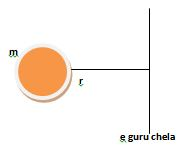# Moment of inertia

Newton's second law, Force = mass x acceleration, relates the acceleration that an object of a certain mass experiences when subject to a given force. There is an analogous relation between torque and angular acceleration, which introduces the concept of moment of inertia: A body maintains the current state of motion unless acted upon by an external force." The measure of the inertia in the linear motion is the mass of the system and its angular counterpart is the so-called moment of inertia. The moment of inertia of a body is not only related to its mass but also the distribution of the mass throughout the body. So two bodies of the same mass may possess different moments of inertia.

$$I = mr^2$$ where m = the mass of the particle, and r = the shortest distance from the axis of rotation to the particle